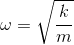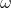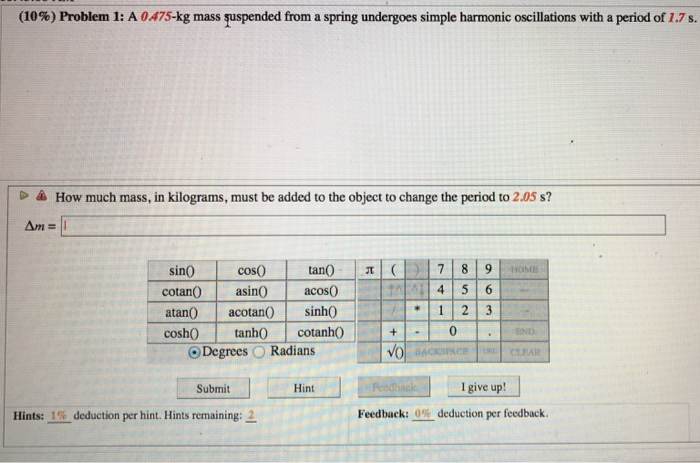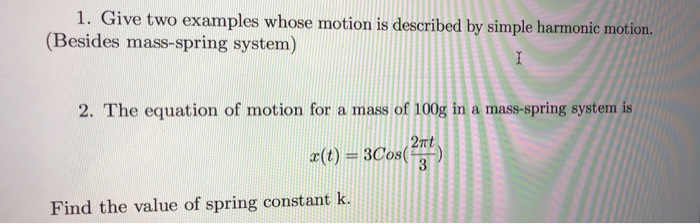# Think of a spring-mass system. Large oscillations of this system _________________________ simple harmonic; small oscillations of...

Think of a spring-mass system. Large oscillations of this system _________________________ simple harmonic; small oscillations of this system ____________________ simple harmonic.

are; are not

are not; are

are not; are not

are; are

Ans:

Since, The frequency of simple harmonic oscillation depends on the spring constant (k) of spring and mass (m) of the object, is given byHence, For higher oscillations (), k should be large and for smaller oscillations (), k should be small or mass (m) can be large.

Hence, large oscillations of spring-mass system are simple harmonic and small oscillations of spring-mass system are simle harmonic.

##### Add Answer of: Think of a spring-mass system. Large oscillations of this system _________________________ simple harmonic; small oscillations of...
Similar Homework Help Questions
• ### Think of a spring-mass system. Large oscillations of this system _________________________ simple harmonic; small oscillations of...

Think of a spring-mass system. Large oscillations of this system _________________________ simple harmonic; small oscillations of this system ____________________ simple harmonic a.are not; are not b.are not; are c.are; are d.are; are not

• ### Frequency and period of simple harmonic oscillations

You pull the 0.5-kg hanging mass 10 cm down from its equilibrium position. When you release it, it starts oscillating to &fro.1)Calculate the characteristic frequency of its natural oscillations. (round the answer to the whole number). Stiffness constant of the spring, k = 20 N/m.Hint: use the formula for natural frequency of simple harmonic oscillations, f. P.S. This frequency is called natural because if you disturb the mass from equilibriumand let it move by itself, its oscillations will be NATURAL.2)...

• ### A block-spring system undergoes simple harmonic motion with an amplitude A. 3.1 If the mass is...

A block-spring system undergoes simple harmonic motion with an amplitude A. 3.1 If the mass is doubled but the amplitude remains unchanged, how will this affect the total energy of the system? 3.2 Can the displacement and the acceleration of the mass be In the same direction? Explain.

• ### A simple harmonic oscillator consists of a block of mass 2.60 kg attached to a spring of spring...

A simple harmonic oscillator consists of a block of mass 2.60 kg attached to a spring of spring constant 178 N/m. When t = 4.00 s, the position and velocity of theblock are x = +0.129 m and v = +3.415 m/s.(a) What is the amplitude of the oscillations?(b) What was the position of the mass at t = 0 s?x = m(c) What was the velocity of the mass at t = 0 s?v = m/s

• ### (10%) Problem 1: A 0475-kg mass suspended from a spring undergoes simple harmonic oscillations with a...(10%) Problem 1: A 0475-kg mass suspended from a spring undergoes simple harmonic oscillations with a period of 1.7 s. How much mass, in kilograms, must be added to the object to change the period to 2.05 s? tan() |π|( acos0 sin0 cos0 cotan 1 2 3 0 atan0 acotansinhO coshO tanh0 cotanhO O Degrees O Radians END Submit Hint I give up! Hints: 1% deduction per hint. Hints remaining Feedback:--deduction per feedback.

• ### A simple harmonic oscillator consists of a block of mass 1.60 kg attached to a spring of spring c...

A simple harmonic oscillator consists of a block of mass 1.60 kg attached to a spring of spring constant 170 N/m. When t = 1.50 s, the position and velocity of the block are x = 0.126 m and v = 3.090 m/s. (a) What is the amplitude of the oscillations? What were the (b) position and (c) velocity of the block at t = 0 s?

• ### A mass/spring system undergoes simple harmonic motion with frequency 1.25 Hz and amplitude .185 meters. If...

A mass/spring system undergoes simple harmonic motion with frequency 1.25 Hz and amplitude .185 meters. If the value of the mass is 167 grams, what is a) the maximum velocity of the mass, and b) what is the total energy in the system?

• ### A simple harmonic oscillator consists of a block of mass 3.50 kg attached to a spring...

A simple harmonic oscillator consists of a block of mass 3.50 kg attached to a spring of spring constant 400 N/m. When t = 1.70 s, the position and velocity of the block are x = 0.121 m and v = 4.020 m/s. (a) What is the amplitude of the oscillations? What were the (b) position and (c) velocity of the block at t = 0 s?

• ### 1. Give two examples whose motion is described by simple harmonic motion. (Besides mass-spring system) 2....1. Give two examples whose motion is described by simple harmonic motion. (Besides mass-spring system) 2. The equation of motion for a mass of 100g in a mass-spring system is 2nt x(t) = 3Cos(f 3 Find the value of spring constant k.

• ### A spring-mass system is in simple harmonic motion. How do the period, maximum speed, frequency, and...

A spring-mass system is in simple harmonic motion. How do the period, maximum speed, frequency, and total mechanical energy of the oscillator change after each of the following alterations (up, down or no change): a) Spring constant (k) is increased? b) Amplitude id increased? c) Mass is decreased?

Free Homework App